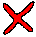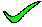To be correct there would have to be a wierd set of determinants in the list. The relevent determinants are a,b->c,d and a,c->b,d. These indicate that we are considering a BCNF problem. Try again.

closeTo be correct there would have to be determinants a->b, b->c, and c->d in the list (and there is not). The relevent determinants are a,b->c,d and a,c->b,d. These indicate that we are considering a BCNF problem. Try again.

closeTo be correct there would have to be determinants a->c, and b->d in the list (and there is not). The relevent determinants are a,b->c,d and a,c->b,d. These indicate that we are considering a BCNF problem. Try again.

closeThis is a valid set of relations, but not as good as the original R. The relevent determinants are a,b->c,d and a,c->b,d. These indicate that we are considering a BCNF problem. Try again.

closeThis is a valid relation, and is identical to the starting relation. The relevent determinants are a,b->c,d and a,c->b,d. These indicate that we are considering a BCNF problem. Every determinant must be a candidate key, so a,b should be able to become the key as well as a,c. Indeed, both can become the key. Another valid relation would therefore be R(a,b,c,d). These relations are in BCNF, and are therefore normalised.

close

 A B C D E TELL ME NEXT INDEX

Consider the following functional dependencies

 a,b => c,d e,g,h => f,j a,c => b,d p,q => r,s e,f,g => h,i s => t f,g => j q => u g,h => i

Which of the following relational schemas might be the result of normalising R(a,b,c,d)?
1. The schema R1(a,b) R2(a,c) R3(a,d)
2. The schema R1(a,b) R2(b,c) R3(c,d)
3. The schema R1(a,b) R2(a,c) R3(b,d)
4. The schema R1(a,b,c) R2(a,b,d)
5. The schema R(a,b,c,d)